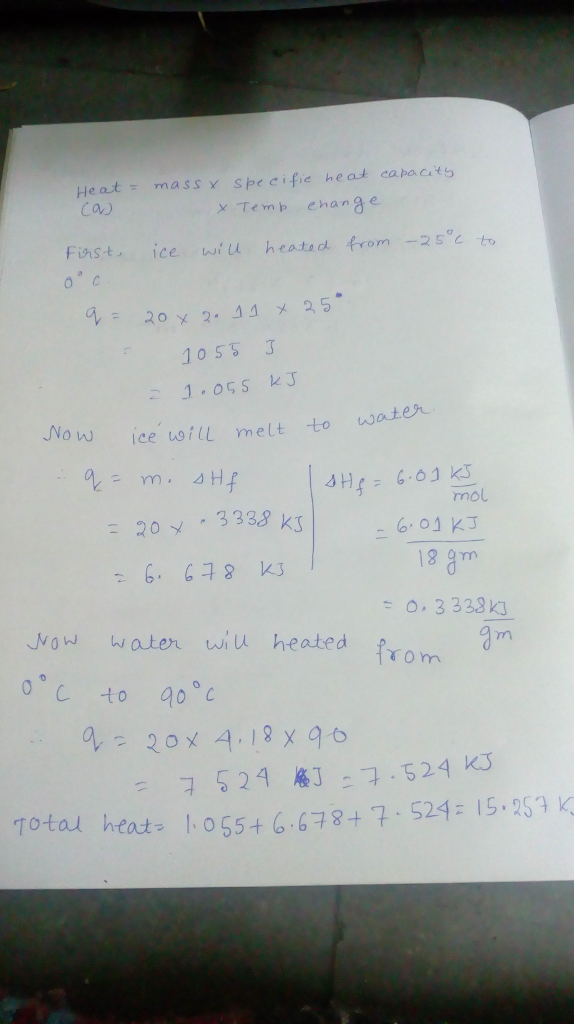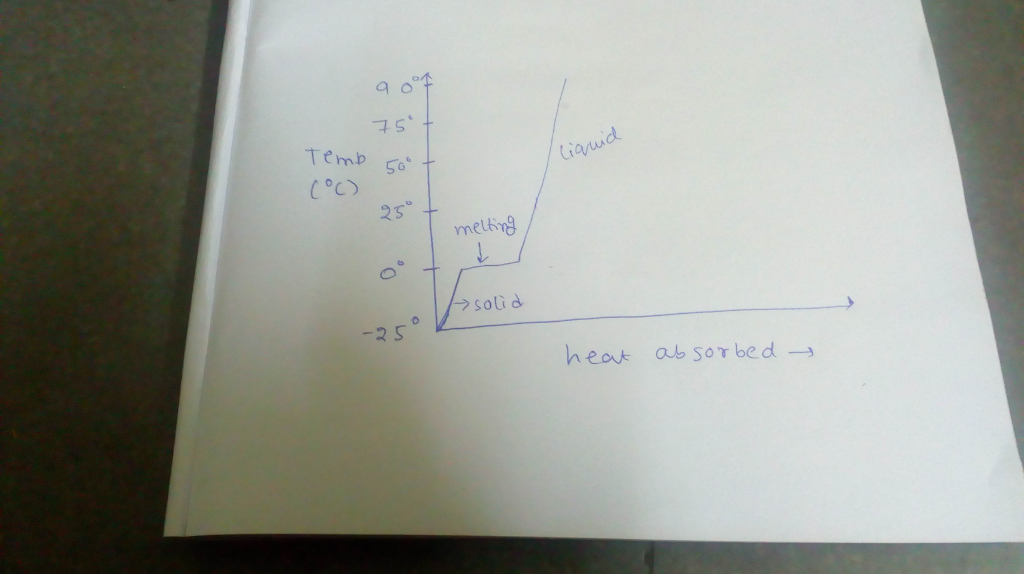# Question & Answer: Calculate the amount of heat in kJ that is required to heat 20.0 g of ice from ?25 degree C to 90…..

Calculate the amount of heat in kJ that is required to heat 20.0 g of ice from ?25 degree C to 90 degree C, and sketch a heating curve for the process. The specific heat of ice is 2.11 J/(g. degree C): water 4.18 J/(g. degree C) and the Delta H_fus for water is 6.01 kJ/mol